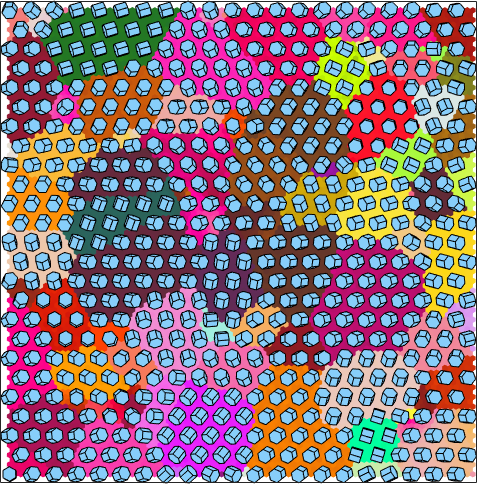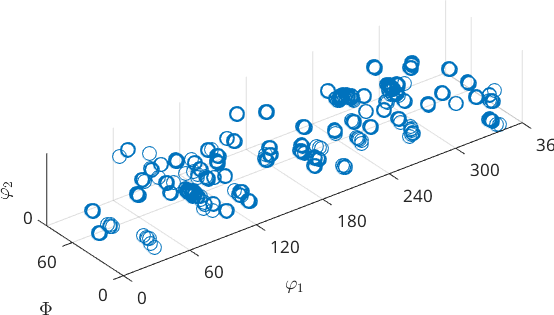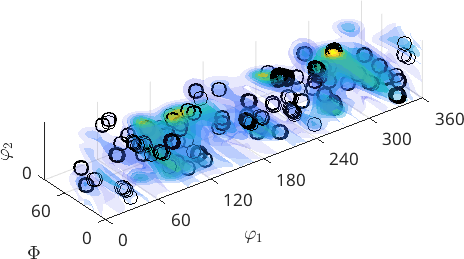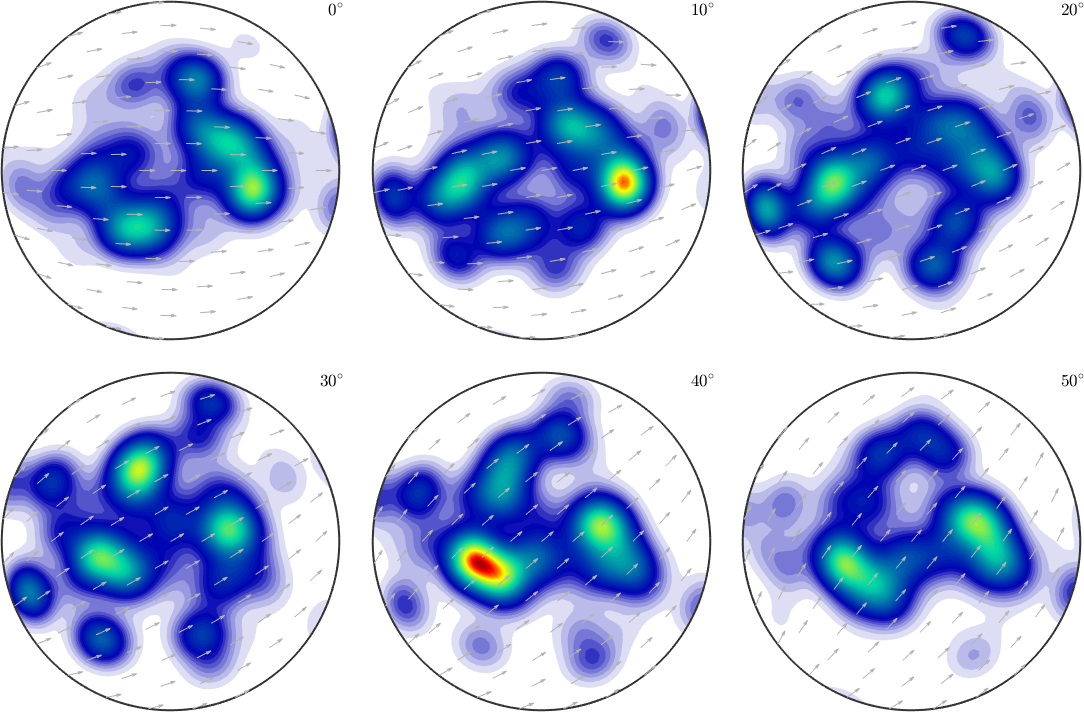The Orientation Distribution Function edit page

The orientation distribution function (ODF), sometimes also called orientation density function, is a function on the orientation space that associates to each orientation $$g$$ the volume percentage of crystals in a polycrystaline specimen that are in this specific orientation. Formaly, this is often expressed by the formula

$\mathrm{odf}(g) = \frac{1}{V} \frac{\mathrm{d}V(g)}{\mathrm{d}g}.$

Let us demonstrate the concept of an ODF at the example of an Titanium alloy. Using EBSD crystal orientations $$g_j$$ have been measured at a hexagonal grid $$(x_j,y_j)$$ on the surface of the specimen. We may visualize these orientations by plotting accordingly rotated crystal shapes at the positions $$(x_j,y_j)$$.The idea of the orientation distribution function is to forget about the spatial coordinates $$(x_j,y_j)$$ and consider the orientations as points in the three dimensional orientation space.As the orientation space is not an Euclidean one there is no canonical way of visualizing it. In the above figure orientations are represented by its three Euler angles $$(\varphi_1, \Phi, \varphi_2)$$. Other visualizations are discussed in the section 3D Plots. The orientation distribution function is now the relative frequency of the above points per volume element and can be computed by the command calcDensity.

More detais about the computation of a density function from discrete measurements can be found in the section Density Estimation.

The resulting orientation distribution function odf can be evaluated for any arbitrary orientation. Lets us e.g. consider the orientation

Then value of the ODF at this orientation is

The resulting value needs to be interpreted as multiple of random distribution (mrd). This means for the specimen under investiagtion it is less likely to have an crystal with orientation (0,0,0) compared to a completely untextured specimen which has the orientation distribution function constant to $$1$$.

Since, an ODF can be evaluated at any point in the orientation space we may visualize it as an contour plot in 3dThree dimensional plot of an ODF in Euler angle space are for various reason not very recommendet. A geometrically much more reasonable representation are so called sigma sections.